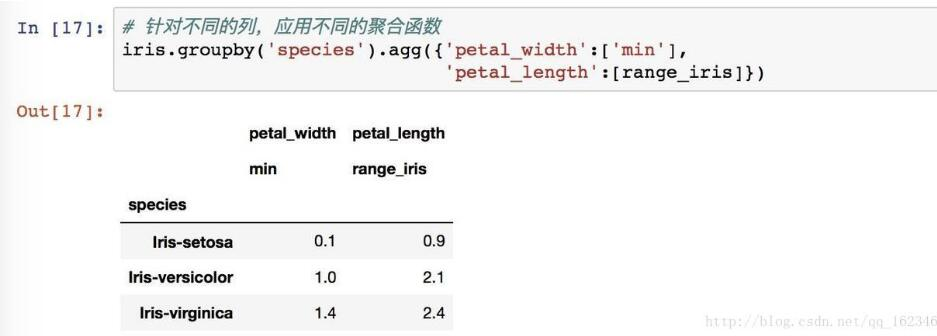# Pandas groupby apply agg 的區別 運行自定義函數說明

agg 方法將一個函數使用在一個數列上，然後返回一個標量的值。也就是說agg每次傳入的是一列數據，對其聚合後返回標量。

## 對一列使用三個函數：## 對不同列使用不同函數apply 是一個更一般化的方法：將一個數據分拆-應用-匯總。而apply會將當前分組後的數據一起傳入，可以返回多維數據。## 實例：

### 1、數據如下：

`lawsuit2[['EID','LAWAMOUNT','LAWDATE']]`### 2、groupby後應用apply傳入函數數據如下：

`lawsuit2[['EID','LAWAMOUNT','LAWDATE']].groupby(['EID']).apply(lambda df:print(df))`### 3、如果使用agg，對於兩列可以處理，但對於上面的三列，打印數據如下：

`lawsuit2[['EID','LAWAMOUNT','LAWDATE']].groupby(['EID']).agg(lambda df:print(df))`### 4、完整代碼：

```def handle(df):
#   print(df)
# 找最大日期
maxdate = df['LAWDATE'].max()
# 找最大費用
left = df[ df['LAWDATE']==maxdate ]['LAWAMOUNT'].max()
# 取ID
EID = df['EID'].values
#   print(EID)
# 從已存在的表中根據EID找到最大費用
right = LAW_AMOUNT_MAX.loc[EID,'LAW_AMOUNT_MAX']
# 判斷費用是否相等
if left==right:
return 1
else:
return 0

LAW_AMOUNT_MAX_IS_LAST = lawsuit2[['EID','LAWAMOUNT','LAWDATE']].groupby(['EID']).apply(handle)```

```orgin = reviews_df.sort_values(["reviewerID","unixReviewTime"]).groupby("reviewerID",group_keys=False)
train = orgin.apply(lambda df: df[:-2])
train.head()```

groupby函數是pandas中用以分組的函數，可以通過指定列來進行分組，並返回一個GroupBy對象。對於GroupBy對象的聚合運算，其有經過優化的較為常用的sum,mean等函數，但是如果我們需要用自定義的函數進行聚合運算，那麼就需要通過agg,apply,transform來實現。

agg,apply和transform三者之間的區別在於：1、agg和transform之間的區別為：前者經過聚合後，隻會在該組單列中返回一個標量值，而transform則會將該標量值在該組單列內進行廣播，保持原DataFrame的索引不變；2、agg和transform中的函數參數是以分組後的單列(Series)為操作對象的，即傳入agg和transform的函數的參數是列，而apply中的函數參數是分組後整個的DataFrame。下面分別對這兩點進行說明。

## 一、agg和transform

```df
Out:
index a b c
0   d 0 1 2
1   d 3 4 5
2   e 6 7 8

df.groupby(by='index').agg(lambda x:x.shape)
Out:
a   b   c
index
d   (2,) (2,) (2,)
e   (1,) (1,) (1,)

df.groupby(by='index').transform(lambda x:x.shape)
Out:
a   b   c
0 (2,) (2,) (2,)
1 (2,) (2,) (2,)
2 (1,) (1,) (1,) ```

## 二、agg和apply

```dd.groupby(by='index').apply(lambda x:x.shape)
Out:
index
d  (2, 4)
e  (1, 4)```